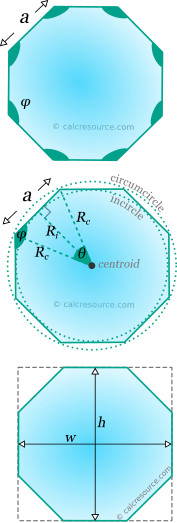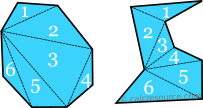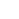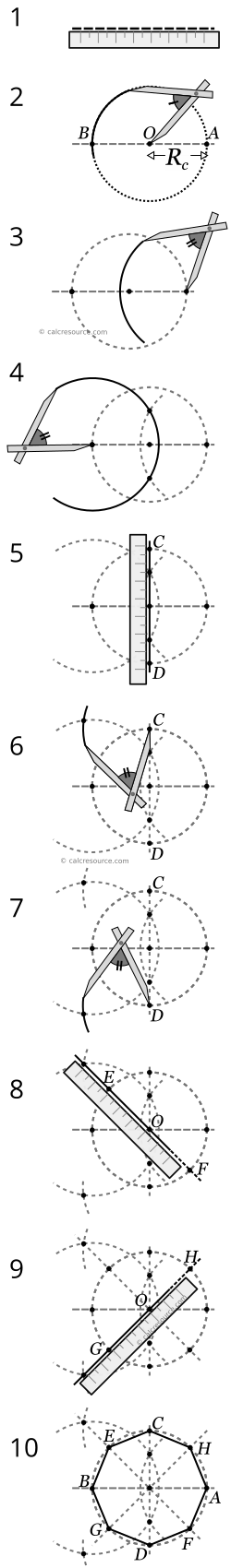## Regular octagon calculator

This tool calculates the basic geometric properties of a regular octagon. Regular polygons are equilateral (all sides equal) and also have all angles equal. The tool can calculate the properties of the octagon, given either the length of its sides, or the inradius or the circumradius or the area or the height or the width. Enter below the shape dimensions. The calculated results will have the same units as your input. Please use consistent units for any input.

 Known data (select): Side length Inradius Circumradius Area Height Width Geometric properties: Area = Perimeter = Edge α = Ri = Rc = Bounding box: Height h = Width w = Angles : deg rad Interior φ = Central θ =## Theoretical background

### Definitions

Octagon is a polygon with eight sides and eight vertices. An octagon, like any other polygon, can be either convex or concave, as illustrated in the next figure. A convex octagon has none of its interior angles greater than 180°. To the contrary, a concave octagon (or polygon) has one or more of its interior angles greater than 180°. An octagon is called regular when its sides are equal and also its interior angles are equal. Having only the sides equal is not adequate, in that matter, since the octagon may be concave, with equal sides. As demonstrated in the figure below, many possible concave octagons can be defined, with equal sides, but unequal interior angles. These octagons are called equilateral. Any octagon that is not regular is called irregular.

The sum of the internal angles of any octagon, either convex or concave is always 1080°. This can be easily be concluded by finding out how many triangles can be fitted inside the octagon. To do so, we have to connect all vertices through straight lines, avoiding any intersections. This way, exactly six triangles can be defined inside the octagon. The vertices can be connected in different ways (resulting in different triangles), however the number of triangles remains constantly six. Since the sum of internal angles in one triangle is 180°, it is concluded that 6 triangles, side by side, should measure up to 6x180=1080°.The diagonals of an octagon separate its interior into 6 triangles

### Properties of regular octagons

#### Symmetry

The regular octagon features eight axes of symmetry. Half of them pass through diagonally opposite vertices and the remaining ones, pass through the midpoints of opposite edges.

#### Interior angle and central angle

Regular octagons have equal interior angles by definition. Since there are eight of them, in total, it can be concluded that each interior angle should be equal to:

The central angle , is the angle between two successive diagonals of the regular octagon (that is lines connecting opposite vertices). There are eight central angles, totally, around a common center of the octagon (where all the diagonals are met), therefore each one should be:

The remaining two angles in the internal triangle, that is formed by two successive diagonals and the side of octagon, are equal to 67.5°. These are indeed , because the diagonals of the octagon are also axes of symmetry, thus bisecting interior angle (which is 135°).

It is worthy to mention, that the interior and central angles are supplementary, since their sum is 180°:The regular octagon is composed by eight identical isosceles triangles having a common vertex, the polygon centre.

#### Circumcircle and incircle

Like any regular polygon, a circle can be drawn that passes through all eight vertices of the octagon. This is the cirmuscribed circle or circumcircle. The center of this circle is the center of the octagon. Likewise, the diagonals of the octagon are diameters of the circumcircle. The radius of circumcircle is usually called circumradius.

Another circle can also be drawn, that passes through the midpoints of the octagon edges. This circle is called inscribed circle or incircle. The radius of incircle is usually called inradius. The incircle is tangent to all eight edges and its center is the same with that of the circumcircle.

The radius of circumcircle, , and incircle, , are related to the length of the edges, . These relationships can be discovered using the right triangle, with sides: the circumradius, the inradius and half the octagon edge, which is shown in the next figure. Using basic trigonometry we may find:

where the central angle and the side length. It turns out that these expressions are valid for any regular polygon, not just the octagon. Specific expressions for the regular octagon can be derived by setting θ = 45°, the value of the central angle. These expressions are:

.

Using the following trigonometric constants for the sine, cosine and tangent:

the equations for the circumradius and inradius of the regular octagon can be expressed in this alternative form:

Approximate expressions for the circumradius and inradius of the regular octagon are given below:

#### Area and perimeter

The total area of a regular octagon can be divided into 8 identical isosceles triangles, as indicated in the figure below. The height of any of these triangles, perpendicular to the octagon edge , is indeed a radius of the incircle, therefore its length is equal to . Therefore, the area of each triangle is , and as a result, the total area of the eight triangles becomes:

Alternatively, using the trigonometric constant , the octagon area can be expressed this way:

An approximate expression for octagon area is:

The perimeter of any N-sided regular polygon is simply the sum of the lengths of all edges: . Therefore, for the regular octagon, with 8 edges:

### Bounding box

The bounding box of a planar shape is the smallest rectangle that encloses the shape completely. The dimensions of this rectangle are defined by the height and the width . The following drawing illustrates the bounding box of a regular octagon. Both the height and the width are defined as the distance between two opposite edges. Due to symmetry, height and width are equal, and as a result the bounding box is actually a square.

##### Height and width

The height and width of the regular octagon are equally defined as the distance between two opposite edges. This length is equal to twice the inradius :

Using the respective analytical expressions for inradius (see previous section) we get:

which is approximated to:

### How to draw a regular octagon

You can draw a regular octagon, given its circumradius , using only a ruler and a compass. Follow the steps described below:

1. First just draw a line (a bit larger than the wanted diameter of the circumcircle).
2. On that line mark two points: one is the center of the polygon (point O), and the other is a point of its circumcircle (point A). Draw a circle around the center point, with radius, , the circumradius. This is the circumcircle. Mark another point where the circumcircle intersects with the line (point B).
3. Then construct a circular arc, having its center at point A, and radius a bit larger than .
4. Also a circular arc, having its center at point B, and same radius with step 3. Mark the two points, where the two arcs intersect. Hint: extent the second arc quite beyond the intersection points.
5. Draw a line, passing through the intersections of the two arcs from the last step (also passing through the center point O) and mark two more points, C and D, where this line intersects with the circumcircle (drawn at step 2).
6. Using the same radius, as in step 4, place the tip of the compass on point C and construct a new circular arc, marking its intersection with the circumcircle.
7. Repeat the same procedure, this time placing the tip at point D, and marking a new intersection point with the circumcircle.
8. Draw a line, passing through the intersection point marked in step 6 and the center point O. Mark the two points, E and F, where this line intersects with the circumcircle.
9. Draw a line, passing through the intersection point marked in step 7 and the center point O. Mark the two points, G and H, where this line intersects with the circumcircle.
10. Eight points have been defined so far around the circumcircle, A, B, C, D, E, F, G, H. These are the vertices of the octagon. Draw linear segments between them and the regular octagon is now complete.

The following figure illustrates the drawing procedure step by step. Note, that compass opening remains the same from step 3 and forward. There is only a change (increase) between steps 2 and 3.Drawing a regular octagon using a ruler and a compass. Note, that the compass opening changes only between steps 2 and 3.

### Examples

##### Example 1

Determine the circumradius, the inradius and the area of a regular octagon with side length .

The circumradius and the inradius, in terms of the side length , for the regular octagon, have been derived in the previous sections. They are:

Therefore, we have to simply substitute . Doing so we find the circumradius:

The area of a regular octagon is given by the equation:

Substituting we find:

##### Example 2

What is the edge length of the following regular octagons:

1. having area
2. having height
##### 1. Regular octagon with given area

The area of a regular octagon , in terms of the side length , is given by the equation:

Since we are looking for , we have to rearrange the formula:

From the last equation we can calculate the required side length , if we substitute :

(To solve this problem we could perfectly use the equivalent expression for the area of the octagon: ).

##### 2. Regular octagon with given height/width

The height (and the width) of the regular octagon is related to the side length with the equation:

Rearranging:

Then we can calculate the required side length , if we substitute :

(Alternatively, we could perfectly use the equivalent expression for height: ).

##### 3. Regular octagon with given circumradius

The circumradius of the regular octagon is related to the side length with the formula:

Therefore:

From the last equation we can calculate the required side length , if we substitute :

(Alternatively, we could perfectly use the equivalent expression for circumradius: ).

### Regular octagon cheat-sheet

In the following table a concise list of the main formulas, related to the regular octagon is included. Also some approximations that may prove handy for practical problems are listed too.